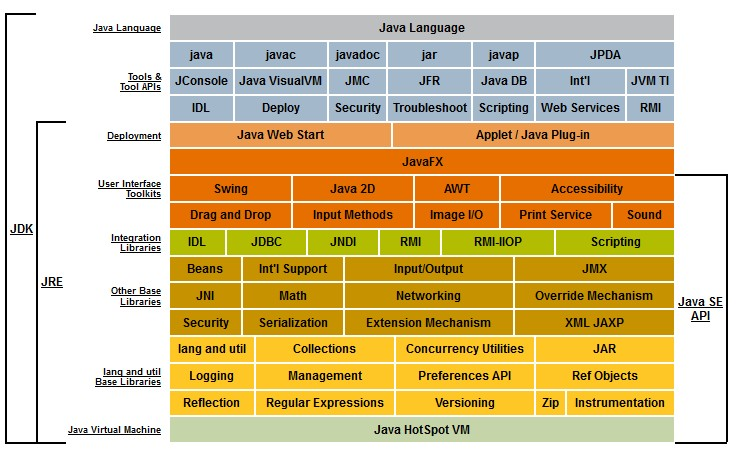# Number - (Arithmetical | Numerical | Mathematical) Operators

Number operators

## Elementary

$addend + addend = sum$

x=1
x+=1
print x
# 2


### Subtraction (−)

$minuend − subtrahend = difference$

Subtraction (-)

### Division (÷ / )

Division (/)

dividend ÷ divisor = quotient


Python:

>>> 3/2
1.5


### Multiplication (×)

$multiplicand × multiplier = product$

## Integer

### Integer Division (Truncating)

Python:

>>> 3//2
1


### Modulo

count_to = 1 + 2


## Expo / Log

### Exponentiation

Exponentiation is a mathematical operation involving two numbers, the base (b) and the exponent (n) (or index or power).

$base^{exponent} = power$

$b^n = power$

Exponentiation (**).

### Logarithm

$log_{base}(power) = exponent$

## Increment/ decrement

• ++: increment
• decrement

Discover MoreJava - Integer

integer data type in Java. int (or Integer) is the 32 bit implementation of an integer. Java can also store an integer on 64 bit with a long. An integer in java is a sub-class of number They are...Java - long - Long (integer 64 bit)

In Java, the long data type stores integer on 64 bit while the integer data type stores integer on 32bit. The primitive wrapper java/lang/LongLong is a subclass of java/lang/NumberNumber in java ...
Javascript - Number (Double)

Most programming languages have several types of numeric data, but JavaScript gets away with just one. All numbers are and are stored as doubles. See also': 32 bit 53 bit - the max of double-precision...Language - Assignment

An assignment is an expression that gives a value to a variable. assignmentstatementexpression assignmentstatementassignment In its simplest form, an assignment statement has a variable on the...Language - Operator

An operator is a symbol (token) that compose an expression. It operates on values on its side (left and/or right). They represents a function but where the argument are on both side of it. This...Mathematics - Exponentiation (square, cube) - Power

Exponentiation is a binary operation involving two numbers: the base (b) the exponent (n) (or index or power). In text notation or computer language, generally the exponentiation operator...Number - Field

A field is a collection of “numbers” with the operator: +, -, , / Different fields are like different classes obeying to the same interface. Same as ?? A field is also known as abody: corps...Ordinal Data - Comparison Operator (Order, Equality)

A comparison operator is a class of operators that model: a order or a equivalence relationship A comparison operator test the order relationship between two operands and returns a Boolean. The...